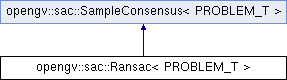OpenGV A library for solving calibrated central and non-central geometric vision problemsopengv::sac::Ransac< PROBLEM_T > Class Template Reference

`#include <Ransac.hpp>`

Inheritance diagram for opengv::sac::Ransac< PROBLEM_T >:## Public Types

typedef PROBLEM_T problem_t

typedef problem_t::model_t model_tPublic Types inherited from opengv::sac::SampleConsensus< PROBLEM_T >
typedef problem_t::model_t model_t

## Public Member Functions

Ransac (int maxIterations=1000, double threshold=1.0, double probability=0.99)
Constructor.

virtual ~Ransac ()
Destructor.

bool computeModel (int debug_verbosity_level=0)
Fit the model.Public Member Functions inherited from opengv::sac::SampleConsensus< PROBLEM_T >
SampleConsensus (int maxIterations=1000, double threshold=1.0, double probability=0.99)
Constructor. More...

virtual ~SampleConsensus ()
Destructor.Public Attributes inherited from opengv::sac::SampleConsensus< PROBLEM_T >
EIGEN_MAKE_ALIGNED_OPERATOR_NEW typedef PROBLEM_T problem_t

int max_iterations_

int iterations_

double threshold_

double probability_

model_t model_coefficients_

std::vector< int > model_

std::vector< int > inliers_

std::shared_ptr< PROBLEM_T > sac_model_

## Detailed Description

### template<typename PROBLEM_T> class opengv::sac::Ransac< PROBLEM_T >

The Ransac sample consensus method, as outlined in .

## Member Typedef Documentation

template<typename PROBLEM_T >
 typedef problem_t::model_t opengv::sac::Ransac< PROBLEM_T >::model_t

The model we trying to fit

template<typename PROBLEM_T >
 typedef PROBLEM_T opengv::sac::Ransac< PROBLEM_T >::problem_t

The documentation for this class was generated from the following file: